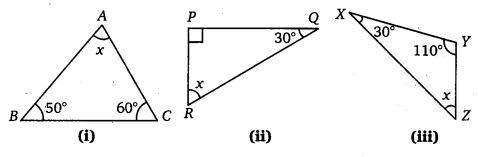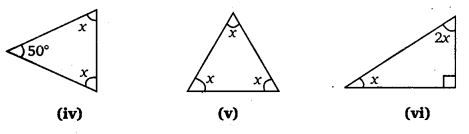# Class 7 Maths NCERT Solutions for Chapter – 6 The Triangle and its Properties Ex – 6.3

### The Triangle and its Properties

Question 1.
Find the value of the unknown x in the following diagrams :Solution:

Question 2.
Find the values of the unknowns x and y in the following diagrams :Solution: